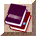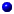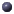##class Random

### Public Methodsstatic double exponential()static double exponential(double r)static int integer(int k)static double lognormal(double avg, double std)static double normal(double avg, double std)static double pareto(double scale, double shape)static double paretoII(double scale, double shape)static int random()static void seed(int s)static int seed_heuristically()static double uniform(double a, double b)static double uniform(double r)static double uniform()

### Private Methodsstatic RNG* rng()

## Documentationstatic RNG* rng()static void seed(int s)static int seed_heuristically()static int random()static double uniform()static double uniform(double r)static double uniform(double a, double b)static double exponential()static int integer(int k)static double exponential(double r)static double pareto(double scale, double shape)static double paretoII(double scale, double shape)static double normal(double avg, double std)static double lognormal(double avg, double std)

This class has no child classes.